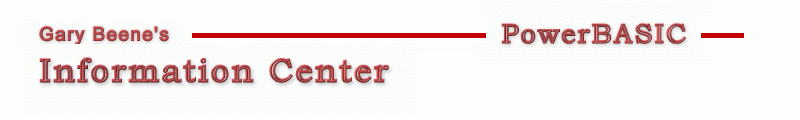## Fit String To Area (Graphic Control)

Category: Strings

Date: 03-28-2012

'Often a programmer needs to size text (change the font) so that it fills
'an area as much as possible. Since there is no Windows API to return a font
'given an area to fill, programmers have to provide their own code.

'Primary Code:
'Credit: Paul Dixon
'Here's an approximation approach to getting the font size that will fill any area. This has
fSize& = IIF( w<h, 0.2*w, 0.2*h)

'However, the above equation gives limited results. This second approach, which assumes the
'font size will be no larger than 1000pts, gives a much better answer - and is very fast!
Function GetFontSize_Graphic2(w As Long, h As Long, txt\$, scalefactor As Single, fontName\$) As Long
Local x As Long, y As Long
Graphic Font fontName\$, 1000, 1
Graphic Text Size txt\$ To x,y
Function=  1000/IIF( x/w > y/h , x/(w*scalefactor) , y/(h*scalefactor) )
End Function

'This following function gives an exact answer by looping through font sizes until a font
'size is found that just fits the given text in the area.  The drawback to this is speed -
'as a result of looping action.
Function GetFontSize_Graphic3(w As Long, h As Long, txt\$, factor As Single, fName\$) As Long
Local x As Long, y As Long, fS&
Do Until x > factor * w Or y > factor * h
Incr fS&
Graphic Font fName\$, fS&, 1
Graphic Text Size txt\$ To x,y
Loop
Function = fS&
End Function

'Finally, here's another exact solution, but this time using a binary search routine to
'minimize the number of loops required to find the answer.
Function GetFontSize_Graphic4(w As Long, h As Long, txt\$, factor As Single, fName\$) As Long
Local x As Long, y As Long, fSize As Long, Upper As Long, Lower As Long
Lower = 1 : Upper = 1000
Do Until (Upper <= (Lower + 1))
fSize = (Lower + Upper) / 2
Graphic Font fName\$, fSize, 1
Graphic Text Size txt\$ To x,y
If (x < factor*w) AND (y < factor*h) Then
Lower = fSize       'fits inside
Else
Upper = fSize      'goes outside
End If
Loop
Function = Lower
End Function

'Compilable Example:
#Compiler PBWin 9, PBWin 10
#Compile EXE
#Dim All
#Include "win32api.inc"
Global hDlg As Dword

Function PBMain () As Long
Local w As Long, h As Long
Desktop Get Client To w, h
Dialog New Pixels, 0, "Control Resize",100,100,200,200, %WS_OverlappedWindow To hDlg
Control Add Graphic, hDlg, 300,"", 0,0,w,h, %WS_Visible Or %SS_Sunken
Graphic Attach hDlg, 300, Redraw
Dialog Show Modal hDlg Call DlgProc
End Function

CallBack Function DlgProc() As Long
Select Case CB.Msg
Case %WM_Size
Dim w As Long, h As Long, x As Long, y As Long, txt\$, fSize&, fName\$
Dialog Get Client CB.Hndl To w,h
Control Set Size CB.Hndl, 300, w-20, h-20
txt\$ = "Sample Text"
fName\$ = "Comic MS Sans"
'get fontsize
fSize& = GetFontSize_Graphic4(w, h, txt\$, 0.9, fName\$)
'center and print
Graphic Clear
Graphic Font fName\$, fSize&, 1
Graphic Text Size txt\$ To x,y
Graphic Set Pos ((w-x)/2,(h-y)/2)
Graphic Print txt\$
Graphic Redraw
End Select
End Function

Function GetFontSize_Graphic2(w As Long, h As Long, txt\$, factor As Single, fontName\$) As Long
Local x As Long, y As Long
Graphic Font fontName\$, 1000, 1
Graphic Text Size txt\$ To x,y
Function=  1000/IIF( x/w > y/h , x/(w*factor) , y/(h*factor) )
End Function

Function GetFontSize_Graphic3(w As Long, h As Long, txt\$, factor As Single, fName\$) As Long
Local x As Long, y As Long, fS&
Do Until x > factor * w Or y > factor * h
Incr fS&
Graphic Font fName\$, fS&, 1
Graphic Text Size txt\$ To x,y
Loop
Dialog Set Text hDlg, Str\$(fS&)
Function = fS&
End Function

Function GetFontSize_Graphic4(w As Long, h As Long, txt\$, factor As Single, fName\$) As Long
Local x As Long, y As Long, fSize As Long, Upper As Long, Lower As Long
Lower = 1 : Upper = 1000
Do Until (Upper <= (Lower + 1))
fSize = (Lower + Upper) / 2
Graphic Font fName\$, fSize, 1
Graphic Text Size txt\$ To x,y
If (x < factor*w) AND (y < factor*h) Then
Lower = fSize       'fits inside
Else
Upper = fSize      'goes outside
End If
Loop
Function = Lower
End Function

'gbs_00360
'Date: 03-10-2012

created by gbSnippets
http://www.garybeene.com/sw/gbsnippets.htm